In AC circuit the emf (e) and the current (i) at any instant are given respectively by

The average power in the circuit over one cycle of AC is

(a)$\frac{{E}_{o}{I}_{o}}{2}$

(b)

(c)

(d) ${E}_{o}{I}_{o}$

High Yielding Test Series + Question Bank - NEET 2020

Difficulty Level:

Power dissipated in an L-C-R series circuit connected to an AC source of emf $\epsilon$ is

(a) $\frac{{\epsilon }^{2}R}{\left[{R}^{2}+{\left(L\omega -\frac{1}{C\omega }\right)}^{2}\right]}$

(b) $\frac{{\epsilon }^{2}{\sqrt{{R}^{2}+\left(L\omega -\frac{1}{C\omega }\right)}}^{2}}{R}$

(c) $\frac{{\epsilon }^{2}\left[{R}^{2}+{\left(L\omega -\frac{1}{C\omega }\right)}^{2}\right]}{R}$

(d) $\frac{{\epsilon }^{2}R}{\sqrt{{R}^{2}+{\left(L\omega -\frac{1}{C\omega }\right)}^{2}}}$

Concept Questions :-

Different types of AC Circuits
High Yielding Test Series + Question Bank - NEET 2020

Difficulty Level:

In the given circuit the reading of voltmeter  are 300V each. The reading to the voltmeter ${V}_{3}$ and ammeter A are respectively :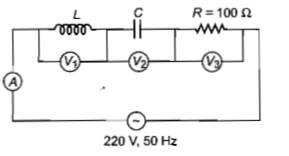(a) 150V, 2.2A                                              (b) 220V, 2.2A

(c) 220V, 2.0A                                              (d) 100V, 2.0A

Concept Questions :-

Different types of AC Circuits
High Yielding Test Series + Question Bank - NEET 2020

Difficulty Level:

A coil has resistance  and inductive reactance  at 50 Hz frequency. If an AC source of 200 V, 100 Hz, is connected across the coil, the current in the coil will be:

1.

2.

3. $\frac{20}{\sqrt{13}}\mathrm{A}$

4.

Concept Questions :-

Different types of AC Circuits
High Yielding Test Series + Question Bank - NEET 2020

Difficulty Level:

The rms value of potential difference V shown in the figure is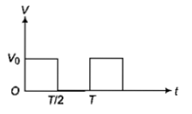(a) ${\mathrm{V}}_{\mathrm{o}}$                                                     (b) $\frac{{\mathrm{V}}_{\mathrm{o}}}{\sqrt{2}}$

(c)$\frac{{\mathrm{V}}_{\mathrm{o}}}{2}$                                                     (d) $\frac{{\mathrm{V}}_{\mathrm{o}}}{\sqrt{3}}$

Concept Questions :-

Rms and average values
High Yielding Test Series + Question Bank - NEET 2020

Difficulty Level:

An AC voltage is applied to a resistance R and an inductor L in series. If R and the inductive reactance are both equal to $3\mathrm{\Omega }$, the phase difference between the applied voltage and the current in the circuit is

(a) $\mathrm{\pi }/4$                                                 (b) $\mathrm{\pi }/2$

(c) zero                                                  (d) $\mathrm{\pi }/6$

High Yielding Test Series + Question Bank - NEET 2020

Difficulty Level:

The current  in a coil varies with time as shown in the figure. The variation of induced emf with time would be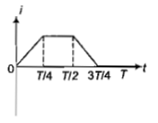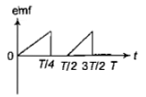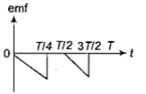(a)                                                                                         (b)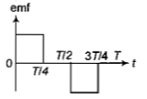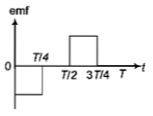(c)                                                                                             (d)

High Yielding Test Series + Question Bank - NEET 2020

Difficulty Level:

In an AC circuit an alternating voltage  volt is connected to a capacitor  The $\mathrm{rms}$ value of the current in the circuit is

(a) 100 mA

(b) 200 mA

(c) 20 mA

(d) 10 mA

High Yielding Test Series + Question Bank - NEET 2020

Difficulty Level:

In a coil of resistance 10$\mathrm{\Omega }$, the induced

current developed by changing magnetic

flux through it is shown in the figure as a

function of time. The magnitude of change

in flux through the coil in weber is-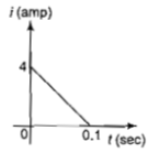(a) 8

(b) 2

(c)6

(d)4

Concept Questions :-

AC vs DC
High Yielding Test Series + Question Bank - NEET 2020

Difficulty Level:

The instantaneous values of alternating

current and voltages in a circuit are given

as

The average power in Watts consumed in the

circuit is

(a) $\frac{1}{4}$

(b) $\frac{\sqrt{3}}{4}$

(c) $\frac{1}{2}$

(d) $\frac{1}{8}$